# Bitcoin Signal Analysis

## Bitcoin Signal Analysis

Updated: Oct 7, 2019

## Introduction

The purpose of this capstone project was to evaluate the efficacy of a neural net-based approach to high-frequency trading in the cryptocurrency market.  The test case considers the Deribit perpetual futures contract limit order book.  Fifty signals were taken as predictors to a binary outcome.  The final model is a Long Short Term Memory (“LSTM”) that achieved an accuracy rate of over 74.

## Background

Trading models must be dynamic and flexible to be successful. Models may rely upon a multitude of variables, both fundamental and technical. In either case, it is well established that an information lag is apparent to either type of predictor – one need only consider the frequent revisions to past-performance indicators to substantiate this claim. It is therefore also apparent that any trading model worth its salt relies upon time-series data, and so is rife the specter of autoregression. Now, the choice of predictors, whether fundamental or technical, comes to the play. We assert that while the technical signal exhibits higher noise than their fundamental counterpart, the underlying predicate to such noise in the form of market activity is of more predictive import. This greater fluidity and exogenous/own time-constraints lead us to base our model on technical indicators alone.

Limit order book data is taken from [April to May 2019] from the Deribit exchange with principal offices in Ermelo, Netherlands.  The choice of exchange was one not strictly of convenience but of prudence; the Deribit exchange is widely regarded as being less subject to the market manipulation that has plagued other exchanges.  The limit order book consists of full order book depth snapshots with incremental updates, tick-by-tick-trades, open interest, funding, liquidations, and quotes.   The perpetual futures contract is a derivative product that is similar to a traditional futures contract but has a few differing specifications to minimize basis, including index-linkage to mimic a margin-based spot market and no expiry.  The Deribit BTC Index, in particular, is measured as the weighted average price of the following six (6) constituents: Bitstamp, Gemini, Bitfinex, Itbit, Coinbase, and Kraken.  Limit orders receive a 0.025% rebate while market orders are charged 0.05%.   The following chart illustrates the LOB data best bid from April 1 – April 15, 2019.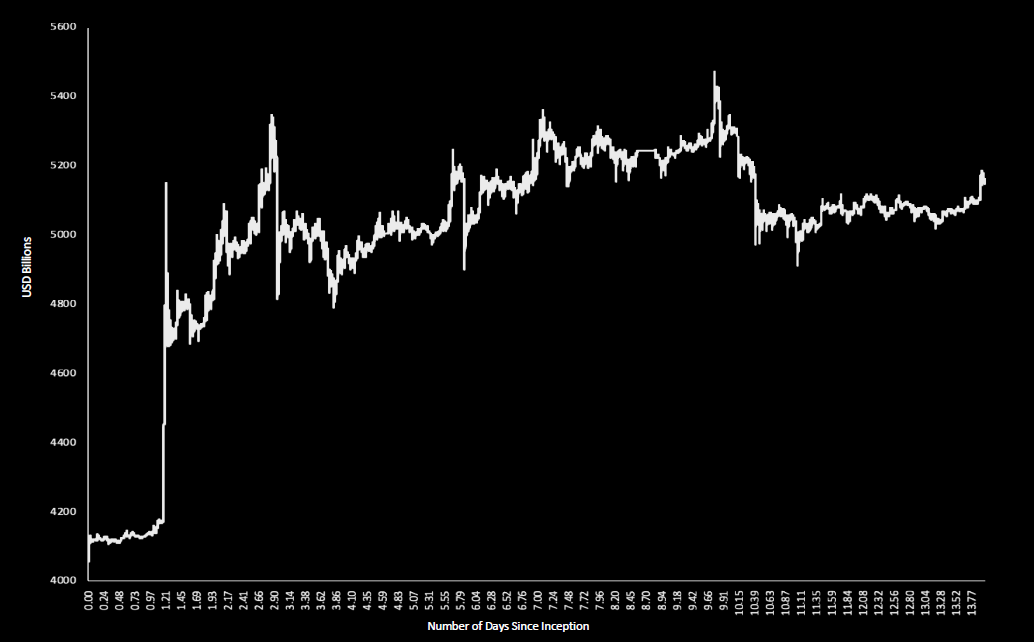The raw form data is, however, inconsistent in time; there are random intervals between observations.  To structure the data that it might be suitable for analysis, a timestamp engineering mechanism was employed whereby data is taken 20-millisecond steps where available and otherwise imputed based on the last value. Subsequently, yield a generator to feed the neural network, aggregate the data over one-minute intervals averaging, summing, or otherwise imputing the data as necessary.

The fifty (50) technical indicators are broadly classifiable by type and include measures of (i) order and return, (ii) trend, (iii) momentum, and (iv) volatility.  Indicators in the main were sourced from the Technical Analysis Library in Python.  Certain indicators were constructed, however, to leverage the data structure of the limit order book.  Examples of such indicators include several cancel orders, volume oscillator(s), and instant volatility.  Source literature is available by request to the authors.   [Detail to be added re lookback, etc.]

## Feature Engineering

### Timestamp Engineering

The raw form data is inconsistent in time and comes in random intervals ranging from 0 to 500 milliseconds between observations. To create consistent data, we tried two different approaches.

Approach 1: 20 Milliseconds Systematically Time Sampling

Our first approach was to sample data every 20 milliseconds, and record in the closest data points. If not a single data point is found within this time-frame, we would copy the last known row of data and repeat it. For our final step, we measured the price difference between the two time-frames, thus creating our Y variable. The data came in the form of a generator that can be directly fed into Keras's Neural Network framework.

However, we soon realized this approach did not perform well across our models because the time difference was too small. Most of the samples had an insignificant change in price. Thus, our model attempted to predict a price change of 0 for nearly all intervals; introducing bias into our prediction.

Approach 2: 1 Minute Time Aggregation

In the next iteration of our project, we decided to aggregate the data over one minute and would either average or sum the data as the signal calculation demands, depending on the feature type. However, this time, since our data-set size has been reduced from a few million rows for one month of data to around 22000, we decided to save the resulting data-set into a CVS file for convenience and general testing purposes. Also, we change our Y from regression to a classification problem; instead of trying to predict the quantity of change, we are just trying to predict the direction of bitcoin price action.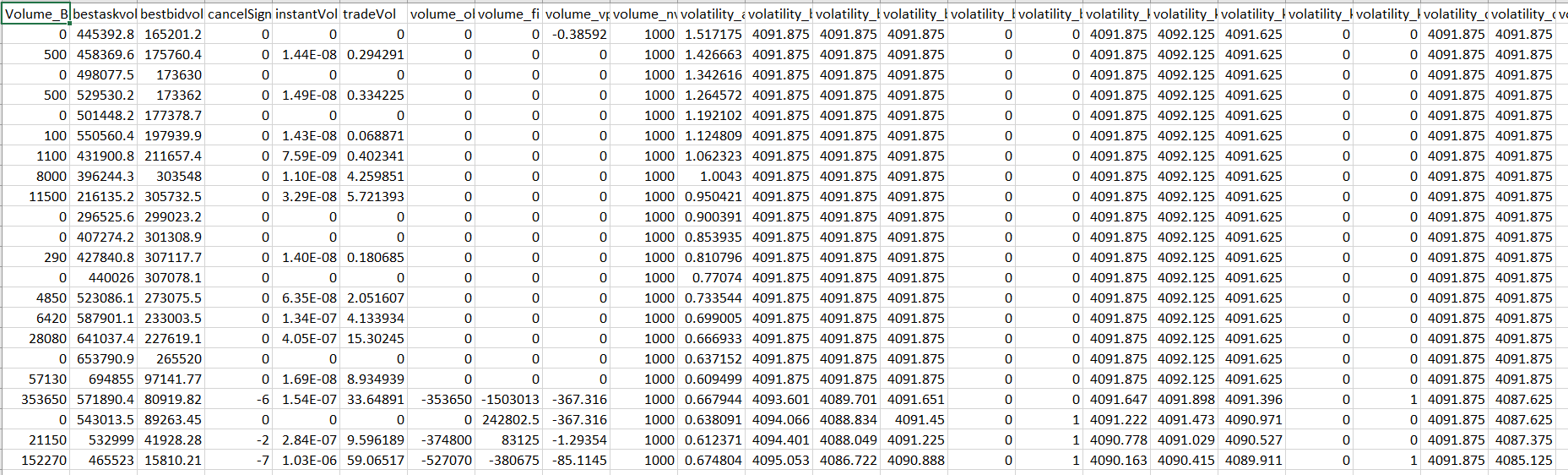### Signal Engineering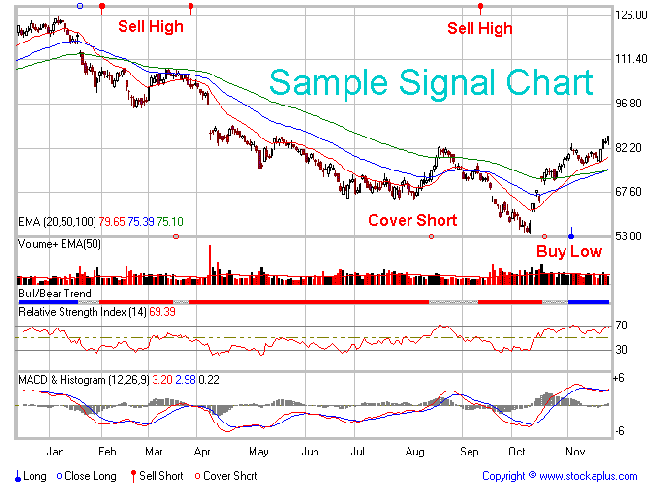Here are some of the signal we use for our program:

#### Volume

• On-Balance Volume (OBV)
• Chaikin Money Flow (CMF)
• Force Index (FI)
• Ease of Movement (EoM, EMV)
• Volume-price Trend (VPT)
• Negative Volume Index (NVI)

#### Volatility

• Instant Volatility (IV)
• Average True Range (ATR)
• Bollinger Bands (BB)
• Keltner Channel (KC)
• Donchian Channel (DC)

#### Trend

• Moving Average Convergence Divergence (MACD)
• Average Directional Movement Index (ADX)
• Vortex Indicator (VI)
• Trix (TRIX)
• Mass Index (MI)
• Commodity Channel Index (CCI)
• Detrended Price Oscillator (DPO)
• KST Oscillator (KST)
• Ichimoku Kinkō Hyō (Ichimoku)

#### Momentum

• Money Flow Index (MFI)
• Relative Strength Index (RSI)
• True strength index (TSI)
• Ultimate Oscillator (UO)
• Stochastic Oscillator (SR)
• Williams %R (WR)
• Awesome Oscillator (AO)
• Kaufman's Adaptive Moving Average (KAMA)

#### Others

• Cancel Signal (CS)
• 15 Interval Return (15IR)
• Daily Return (DR)
• Daily Log Return (DLR)
• Cumulative Return (CR)

## Machine Learning Modeling

### Step 1: Testing Individual Models

#### Base Model: Logistic Regression Classification

For classification problems, the Logistic Regression model is a good base from which we can compare performance. However, it has some limitations:

1. It requires the observations to be independent of each other. In a continuous time-series of a market, this is not the case.
2. Demands little to no multi-collinearity among the X variables. Since most of our signals (i.e. moving average or momentum) were calculated from the same price and volume variables - they are are multicollinear.
3. The X variables need to be linearly related to the log odds.

Since our dataset breaks many of these rules from Logistic Regression, we do not expect this model to perform well. After performing hyperparameters tuning using Bayesian optimization, the confusion matrix for Logistic Regression and the model accuracy is shown below.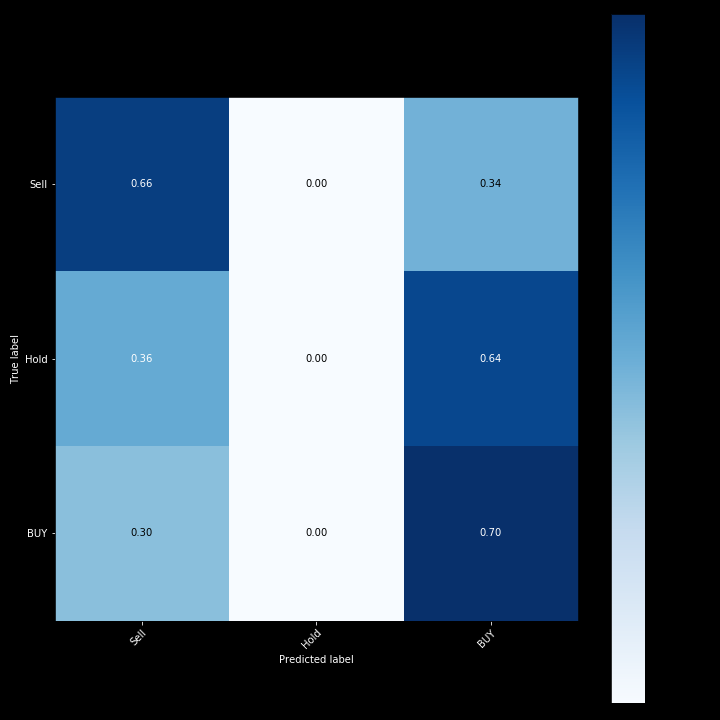Logistic Regression Score of different time period: 0.453 - 0.6496

Below are some outputs for the Logistic Regression Model.

Y_Pred:

• Sell: 12348
• Nothing: 5,

Y_Actual:

• Sell: 15551
• Nothing: 773

As you can see from the result, the LR model was very inconsistent and most of the time, it is slightly better than a random assignment. We decided to use a different model that could handle the non-linearity of our data.

#### Advanced Base Model: Support Vector Machine Classification

The next algorithm we used was SVM, a non-linear, non-parametric classification technique. SVM employs kernel tricks and maximal margin concepts, so in theory, it performs much better in non-linear and high dimensional tasks. However, there is "no free lunch" in using SVM.

Benefits of SVM:

• Flexibility: Capable of fitting a large number of functional forms.
• Power: No assumptions or weak assumptions about the underlying function.
• Performance for complex model: Can result in higher performance models for prediction.

Limitations:

• Time Consuming: Finding the right kernel function is not easy
• Slower: Kernelized SVMs require the computation of a distance function between each point in the dataset, which is the dominating cost of O(n features×n^2 observations) - resulting in long training time for large datasets.
• Overfitting: Higher risk of overfitting on training data and harder to explain predictions.

After performing hyperparameter tuning using Bayesian optimization, the confusion matrix of the SVM classifier and its model accuracy is shown below.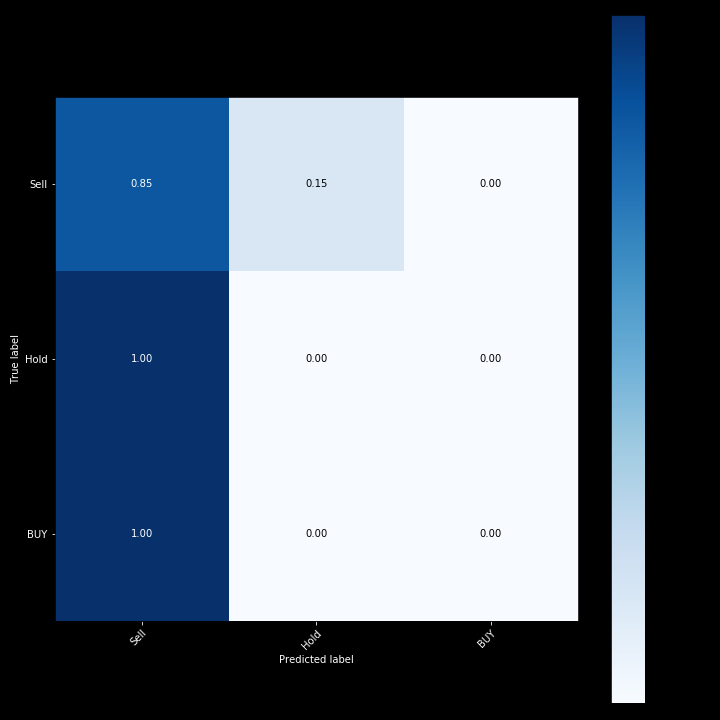SVM Score of different time period: 0.513 - 0.639

Here are some of the outputs for the SVM Model.

Y_Pred:

• Sell: 19904
• Nothing: 2345,

Y_Actual:

• Sell: 15551
• Nothing: 773

The SVM does much better consistently than the LR model, which is not a surprise, however, due to the dataset size increase, our training time increased exponentially, making testing of the different model parameters very unrealistic. Every model would take around 4 to 8 hours to train depending on the kernel type. Due to long training timing, we decided to use a neural network to further increase the performance and handle a large amount of data.

#### Super Advanced Model: Long-Short Term Memory Neural Network

While the models thus far have accomplished some level of accuracy, they’re crucially inadequate for time-series data. We needed a model that could accommodate for time, as well as the nonlinearity and size of our data. Given these constraints, we decided to use a Long Short Term Memory (LSTM) neural network. It is a version of a Recurrent Neural Network (RNN), which feeds previous states into the current one, thus accommodating the timestep nature of our data. One of the primary concerns with an RNN is that its weight matrices change too quickly from state to state, thus losing some underlying meaning beyond a certain period. An LSTM accommodates this deficiency by implementing a ‘permanent memory cell’ – the weight matrix of which is independent from each layer matrix.

We then added a dropout layer using the standard value of 0.2 to discourage overfitting on our training data. A dropout layer omits the desired portion of neurons, at random, during backpropagation. This type of augmentation is called regularization, or the bias-variance tradeoff. We also used l1 regularization on our LSTM layer, which drives the weight matrices towards 0, resulting in an omission of certain variables. Given that markets change their regimes at unknown intervals, we wanted to make sure that our model could handle a test data set with entirely different conditions.

Our final layer was a Dense layer with a softmax activation function. Softmax takes a vector and converts it into a probability distribution, which allows us to interpret the probability of the next direction. We then compiled the model with categorical cross-entropy, which measures and minimizes the log loss between prediction and real output.

We then began training the model. After some tuning, we realized a critical component of our data. Accurately predicting direction (up or down) was more important than predicting that price would stay the same between two periods. Thus, we ended up weighting our classes of [sell, hold, buy] as [1, 0.2, 1], meaning, we drastically reduced the importance of accurately predicting hold. An improvement upon this weighting is to scale it with the volatility of the asset class that is being traded.

When it came to model tuning, we noticed that validation loss increased with time, while validation accuracy dropped. This is symptomatic of having an insufficiently large data set, which is one of the next steps in our project. Our model converged on the training and validation datasets rather quickly (sub-50 epochs), and this concluded with us obtaining 74% average accuracy across a number of runs. We want to note that although this accuracy is high, there are still many steps to take before this black box can be effectively used during execution.

## Future Work

• Build-in additional features and adjust model parameters to capture the impact of exogenous factors and develop model intuition.
• Evaluate liquidity characteristics by exchange including the impact of funding vis-à-vis a thorough analysis of exchange funding algorithms.
• Develop a multi-exchange framework to capture potential arbitrage opportunities.
• Evaluate the nuances of optimizing loss versus accuracy.
• Build additional models including negative affirmation trading, price magnitude sensitivity, and intra-market cross-currency correlation models.